MathScore EduFighter is one of the best math games on the Internet today. You can start playing for free!

## Probability - Sample Math Practice Problems

The math problems below can be generated by MathScore.com, a math practice program for schools and individual families. References to complexity and mode refer to the overall difficulty of the problems as they appear in the main program. In the main program, all problems are automatically graded and the difficulty adapts dynamically based on performance. Answers to these sample questions appear at the bottom of the page. This page does not grade your responses.

See some of our other supported math practice problems.

### Complexity=5

Find the probability that a randomly selected piece of the shape will be highlighted and find the odds that a piece chosen will not be highlighted. Express probabilities as a simplified fraction and odds as a ratio of two numbers with no common factors other than 1 (i.e. "2:1").

1.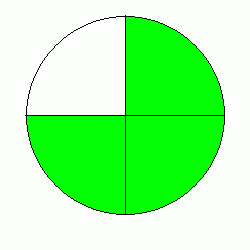Probability: Odds:

2.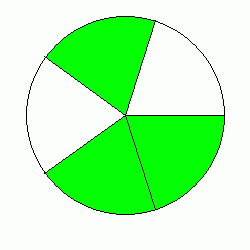Probability: Odds:

### Complexity=6

Find the probability that a randomly selected piece of the shape will be highlighted and find the odds that a piece chosen will not be highlighted. Express probabilities as a simplified fraction and odds as a ratio of two numbers with no common factors other than 1 (i.e. "2:1").

1.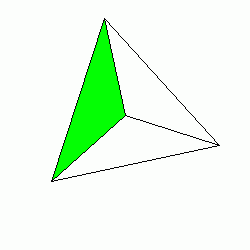Probability: Odds:

2.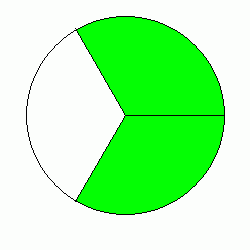Probability: Odds:

### Complexity=7

Find the probability that a randomly selected piece of the shape will be highlighted and find the odds that a piece chosen will not be highlighted. Express probabilities as a simplified fraction and odds as a ratio of two numbers with no common factors other than 1 (i.e. "2:1").

1.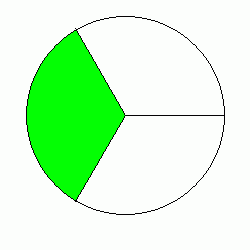Probability: Odds:

2.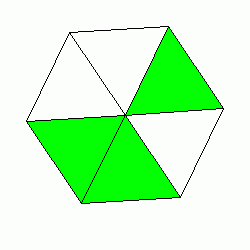Probability: Odds:

### Complexity=8

Find the probability that a randomly selected piece of the shape will be highlighted and find the odds that a piece chosen will not be highlighted. Express probabilities as a simplified fraction and odds as a ratio of two numbers with no common factors other than 1 (i.e. "2:1").

1.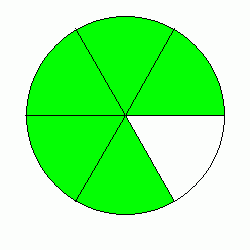Probability: Odds:

2.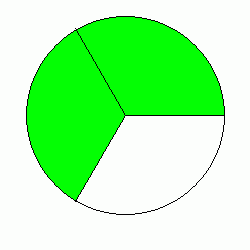Probability: Odds:

### Complexity=9

Find the probability that a randomly selected piece of the shape will be highlighted and find the odds that a piece chosen will not be highlighted. Express probabilities as a simplified fraction and odds as a ratio of two numbers with no common factors other than 1 (i.e. "2:1").

1.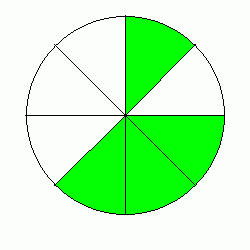Probability: Odds:

2.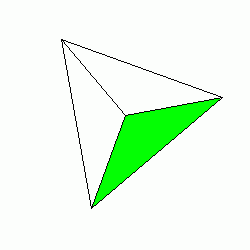Probability: Odds:

### Complexity=10

Find the probability that a randomly selected piece of the shape will be highlighted and find the odds that a piece chosen will not be highlighted. Express probabilities as a simplified fraction and odds as a ratio of two numbers with no common factors other than 1 (i.e. "2:1").

1.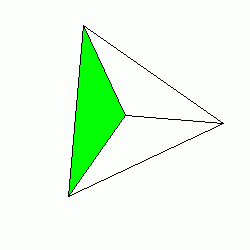Probability: Odds:

2.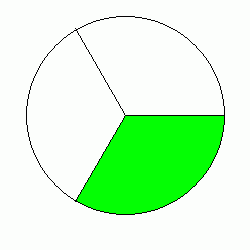Probability: Odds:

### Complexity=5

Find the probability that a randomly selected piece of the shape will be highlighted and find the odds that a piece chosen will not be highlighted. Express probabilities as a simplified fraction and odds as a ratio of two numbers with no common factors other than 1 (i.e. "2:1").

1Probability: Odds:

Solution
There are 3 pieces that are highlighted out of 4 pieces. Thus, the probability that a randomly chosen piece is highlighted is 3/4.

Since odds is a ratio of the event happening to the event not happening, we can simply take a ratio of the number of pieces unhighlighted to the number of pieces highlighted. In this case that would be 1:3.

2Probability: Odds:

Solution
There are 3 pieces that are highlighted out of 5 pieces. Thus, the probability that a randomly chosen piece is highlighted is 3/5.

Since odds is a ratio of the event happening to the event not happening, we can simply take a ratio of the number of pieces unhighlighted to the number of pieces highlighted. In this case that would be 2:3.

### Complexity=6

Find the probability that a randomly selected piece of the shape will be highlighted and find the odds that a piece chosen will not be highlighted. Express probabilities as a simplified fraction and odds as a ratio of two numbers with no common factors other than 1 (i.e. "2:1").

1Probability: Odds:

Solution
There is 1 piece that is highlighted out of 3 pieces. Thus, the probability that a randomly chosen piece is highlighted is 1/3.

Since odds is a ratio of the event happening to the event not happening, we can simply take a ratio of the number of pieces unhighlighted to the number of pieces highlighted. In this case that would be 2:1.

2Probability: Odds:

Solution
There are 2 pieces that are highlighted out of 3 pieces. Thus, the probability that a randomly chosen piece is highlighted is 2/3.

Since odds is a ratio of the event happening to the event not happening, we can simply take a ratio of the number of pieces unhighlighted to the number of pieces highlighted. In this case that would be 1:2.

### Complexity=7

Find the probability that a randomly selected piece of the shape will be highlighted and find the odds that a piece chosen will not be highlighted. Express probabilities as a simplified fraction and odds as a ratio of two numbers with no common factors other than 1 (i.e. "2:1").

1Probability: Odds:

Solution
There is 1 piece that is highlighted out of 3 pieces. Thus, the probability that a randomly chosen piece is highlighted is 1/3.

Since odds is a ratio of the event happening to the event not happening, we can simply take a ratio of the number of pieces unhighlighted to the number of pieces highlighted. In this case that would be 2:1.

2Probability: Odds:

Solution
There are 3 pieces that are highlighted out of 6 pieces. Thus, the probability that a randomly chosen piece is highlighted is 1/2.

Since odds is a ratio of the event happening to the event not happening, we can simply take a ratio of the number of pieces unhighlighted to the number of pieces highlighted. In this case that would be 3:3 which simplifies to 1:1.

### Complexity=8

Find the probability that a randomly selected piece of the shape will be highlighted and find the odds that a piece chosen will not be highlighted. Express probabilities as a simplified fraction and odds as a ratio of two numbers with no common factors other than 1 (i.e. "2:1").

1Probability: Odds:

Solution
There are 5 pieces that are highlighted out of 6 pieces. Thus, the probability that a randomly chosen piece is highlighted is 5/6.

Since odds is a ratio of the event happening to the event not happening, we can simply take a ratio of the number of pieces unhighlighted to the number of pieces highlighted. In this case that would be 1:5.

2Probability: Odds:

Solution
There are 2 pieces that are highlighted out of 3 pieces. Thus, the probability that a randomly chosen piece is highlighted is 2/3.

Since odds is a ratio of the event happening to the event not happening, we can simply take a ratio of the number of pieces unhighlighted to the number of pieces highlighted. In this case that would be 1:2.

### Complexity=9

Find the probability that a randomly selected piece of the shape will be highlighted and find the odds that a piece chosen will not be highlighted. Express probabilities as a simplified fraction and odds as a ratio of two numbers with no common factors other than 1 (i.e. "2:1").

1Probability: Odds:

Solution
There are 4 pieces that are highlighted out of 8 pieces. Thus, the probability that a randomly chosen piece is highlighted is 1/2.

Since odds is a ratio of the event happening to the event not happening, we can simply take a ratio of the number of pieces unhighlighted to the number of pieces highlighted. In this case that would be 4:4 which simplifies to 1:1.

2Probability: Odds:

Solution
There is 1 piece that is highlighted out of 3 pieces. Thus, the probability that a randomly chosen piece is highlighted is 1/3.

Since odds is a ratio of the event happening to the event not happening, we can simply take a ratio of the number of pieces unhighlighted to the number of pieces highlighted. In this case that would be 2:1.

### Complexity=10

Find the probability that a randomly selected piece of the shape will be highlighted and find the odds that a piece chosen will not be highlighted. Express probabilities as a simplified fraction and odds as a ratio of two numbers with no common factors other than 1 (i.e. "2:1").

1Probability: Odds:

Solution
There is 1 piece that is highlighted out of 3 pieces. Thus, the probability that a randomly chosen piece is highlighted is 1/3.

Since odds is a ratio of the event happening to the event not happening, we can simply take a ratio of the number of pieces unhighlighted to the number of pieces highlighted. In this case that would be 2:1.

2Probability: Odds:

Solution
There is 1 piece that is highlighted out of 3 pieces. Thus, the probability that a randomly chosen piece is highlighted is 1/3.

Since odds is a ratio of the event happening to the event not happening, we can simply take a ratio of the number of pieces unhighlighted to the number of pieces highlighted. In this case that would be 2:1.